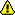You should trust both wikis because the password could be read by the particular administrators.

Clear message

# Name

dilation - Calculate the dilated image (for both binary and graylevel image).

# Usage

output = dilation(input, mask, morphtype = "morph_type")

## Input

input
The first input image
The second input image used as the mask (default: image of size 3^n filled with ones).
• The size of the mask has to be odd so that the center of mask can be well defined.
• The size of the mask should be smaller than the size of the first input image.
morph_type
Type of the dilation
• BINARY is for binary dilation;
• GRAYLEVEL is for graylevel dilation.

output
dilated image

# Method

1. For dilation binary, the output image is defined as the Minkowski addition of the two input images \$A\oplusB={t\inZ^2; t=a+b, a\inA, b\inB}\$
2. For dilation graylevel, the output image is defined as \$A\oplusB=\max[A(x+i,y+j)+B(i,j)]\$

# Reference

H. R. Myler and A. R. Weeks, "The Pocket Handbook of Image Processing Algorithms in C," Prentice Hall: Upper Saddle River, New Jersey, 1993.

Pawel A. Penczek

# Keywords

category 1
MORPHOLOGY
category 2
SPATIAL, NONLINEAR

morphology.py